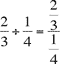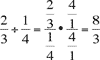# Natasha’s Idea

Natasha suggests that fractions can easily be divided by doing the following:Then she solves it by multiplying the numerator and the denominator by 4/1 to create a denominator of 1:Does this always work? How does it relate to the ‘invert and multiply’ algorithm?